## The Dirac Equation

Our goal is to find the analog of the Schrödinger equation for relativistic spin one-half particles, however, we should note that even in the Schrödinger equation, the interaction of the field with spin was rather ad hoc. There was no explanation of the gyromagnetic ratio of 2. One can incorporate spin into the non-relativistic equation by using the Schrödinger-Pauli Hamiltonianwhich contains the dot product of the Pauli matrices with the momentum operator.A little computation shows that this gives the correct interaction with spin.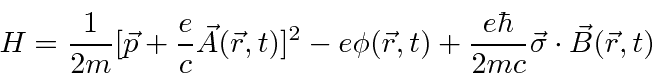This Hamiltonian acts on a two component spinor.

We can extend this concept to use the relativistic energy equation. The idea is to replacewithin the relativistic energy equation.Instead of an equation which is second order in the time derivative, we can make a first order equation, like the Schrödinger equation, by extending this equation to four components.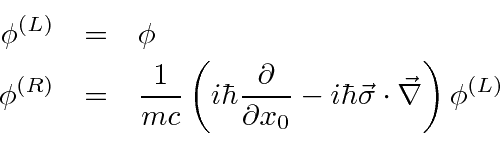Now rewriting in terms ofand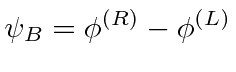and ordering it as a matrix equation, we get an equation that can be written as a dot product between 4-vectors.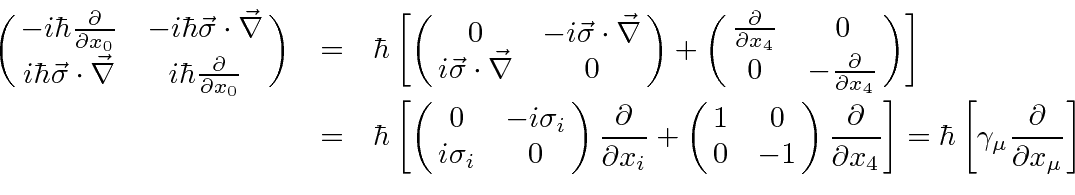Define the 4 by 4 matricesare by.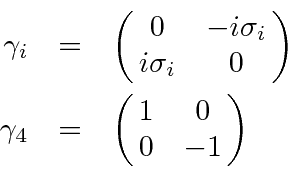With this definition, the relativistic equation can be simplified a great dealwhere the gamma matrices are given by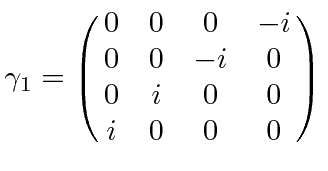and they satisfy anti-commutation relations.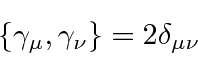In fact any set of matrices that satisfy the anti-commutation relations would yield equivalent physics results, however, we will work in the above explicit representation of the gamma matrices.

Defining,satisfies the equation of a conserved 4-vector current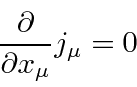and also transforms like a 4-vector. The fourth component of the vector shows that the probability density is. This indicates that the normalization of the state includes all four components of the Dirac spinors.

For non-relativistic electrons, the first two components of the Dirac spinor are large while the last two are small.We use this fact to write an approximate two-component equation derived from the Dirac equation in the non-relativistic limit.This Schrödinger equation'', derived from the Dirac equation, agrees well with the one we used to understand the fine structure of Hydrogen. The first two terms are the kinetic and potential energy terms for the unperturbed Hydrogen Hamiltonian. The third term is the relativistic correction to the kinetic energy. The fourth term is the correct spin-orbit interaction, including the Thomas Precession effect that we did not take the time to understand when we did the NR fine structure. The fifth term is the so called Darwin term which we said would come from the Dirac equation; and now it has.

For a free particle, each component of the Dirac spinor satisfies the Klein-Gordon equation.This is consistent with the relativistic energy relation.

The four normalized solutions for a Dirac particle at rest are.The first and third have spin up while the second and fourth have spin down. The first and second are positive energy solutions while the third and fourth are negative energy solutions'', which we still need to understand.

The next step is to find the solutions with definite momentum. The four plane wave solutions to the Dirac equation arewhere the four spinors are given by.is positive for solutions 1 and 2 and negative for solutions 3 and 4. The spinors are orthogonal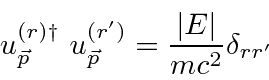and the normalization constants have been set so that the states are properly normalized and the spinors follow the convention given above, with the normalization proportional to energy.

The solutions are not in general eigenstates of any component of spin but are eigenstates of helicity, the component of spin along the direction of the momentum.

Note that withnegative, the exponential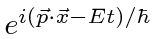has the phase velocity, the group velocity and the probability flux all in the opposite direction of the momentum as we have defined it. This clearly doesn't make sense. Solutions 3 and 4 need to be understood in a way for which the non-relativistic operators have not prepared us. Let us simply relabel solutions 3 and 4 such thatso that all the energies are positive and the momenta point in the direction of the velocities. This means we change the signs in solutions 3 and 4 as follows.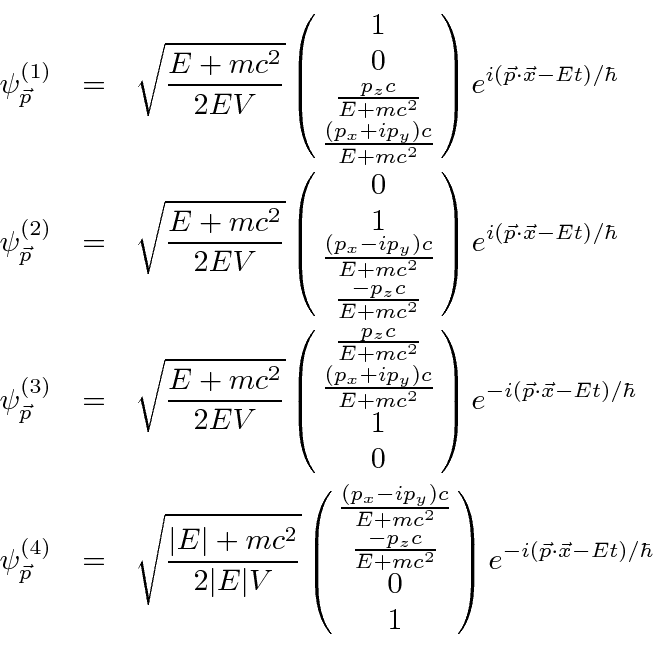We have plane waves of the formwith the plus sign for solutions 1 and 2 and the minus sign for solutions 3 and 4. These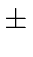sign in the exponential is not very surprising from the point of view of possible solutions to a differential equation. The problem now is that for solutions 3 and 4 the momentum and energy operators must have a minus sign added to them and the phase of the wave function at a fixed position behaves in the opposite way as a function of time than what we expect and from solutions 1 and 2. It is as if solutions 3 and 4 are moving backward in time.

If we change the charge on the electron from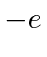toand change the sign of the exponent, the Dirac equation remains the invariant. Thus, we can turn the negative exponent solution (going backward in time) into the conventional positive exponent solution if we change the charge to. We can interpret solutions 3 and 4 as positrons. We will make this switch more carefully when we study the charge conjugation operator.

The Dirac equation should be invariant under Lorentz boosts and under rotations, both of which are just changes in the definition of an inertial coordinate system. Under Lorentz boosts,transforms like a 4-vector but thematrices are constant. The Dirac equation is shown to be invariant under boosts along thedirection if we transform the Dirac spinor according towith.

The Dirac equation is invariant under rotations about the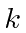axis if we transform the Dirac spinor according to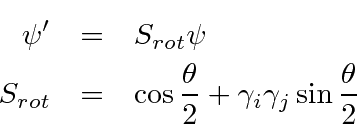withis a cyclic permutation.

Another symmetry related to the choice of coordinate system is parity. Under a parity inversion operation the Dirac equation remains invariant ifSince, the third and fourth components of the spinor change sign while the first two don't. Since we could have chosen, all we know is that components 3 and 4 have the opposite parity of components 1 and 2.

From 4 by 4 matrices, we may derive 16 independent components of covariant objects. We define the product of all gamma matrices.which obviously anticommutes with all the gamma matrices.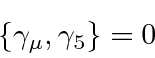For rotations and boosts,commutes with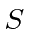since it commutes with the pair of gamma matrices. For a parity inversion, it anticommutes with.

The simplest set of covariants we can make from Dirac spinors andmatrices are tabulated below.

 Classification Covariant Form no. of Components Scalar1 Pseudoscalar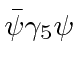1 Vector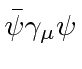4 Axial Vector4 Rank 2 antisymmetric tensor6 Total 16
Products of morematrices turn out to repeat the same quantities because the square of anymatrix is 1.

For many purposes, it is useful to write the Dirac equation in the traditional form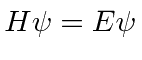. To do this, we must separate the space and time derivatives, making the equation less covariant looking.Thus we can identify the operator below as the Hamiltonian.The Hamiltonian helps us identify constants of the motion. If an operator commutes with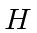, it represents a conserved quantity.

Its easy to see the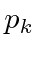commutes with the Hamiltonian for a free particle so that momentum will be conserved. The components of orbital angular momentum do not commute with.The components of spin also do not commute with.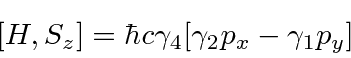But, from the above, the components of total angular momentum do commute with.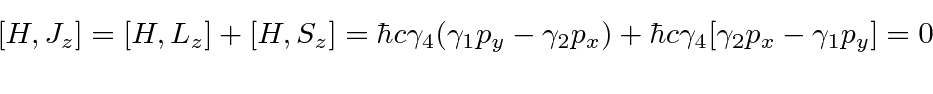The Dirac equation naturally conserves total angular momentum but not the orbital or spin parts of it.

We can also see that the helicity, or spin along the direction of motion does commute.For any calculation, we need to know the interaction term with the Electromagnetic field. Based on the interaction of field with a current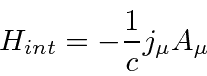and the current we have found for the Dirac equation, the interaction Hamiltonian is.This is simpler than the non-relativistic case, with noterm and only one power of.

The Dirac equation has some unexpected phenomena which we can derive. Velocity eigenvalues for electrons are alwaysalong any direction. Thus the only values of velocity that we could measure are.

Localized states, expanded in plane waves, contain all four components of the plane wave solutions. Mixing components 1 and 2 with components 3 and 4 gives rise to Zitterbewegung, the very rapid oscillation of an electrons velocity and position.The last sum which contains the cross terms between negative and positive energy represents extremely high frequency oscillations in the expected value of the velocity, known as Zitterbewegung. The expected value of the position has similar rapid oscillations.

It is possible to solve the Dirac equation exactly for Hydrogen in a way very similar to the non-relativistic solution. One difference is that it is clear from the beginning that the total angular momentum is a constant of the motion and is used as a basic quantum number. There is another conserved quantum number related to the component of spin along the direction of. With these quantum numbers, the radial equation can be solved in a similar way as for the non-relativistic case yielding the energy relation.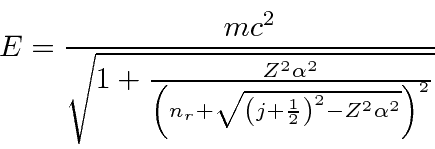We can identify the standard principle quantum number in this case as. This result gives the same answer as our non-relativistic calculation to orderbut is also correct to higher order. It is an exact solution to the quantum mechanics problem posed but does not include the effects of field theory, such as the Lamb shift and the anomalous magnetic moment of the electron.

A calculation of Thomson scattering shows that even simple low energy photon scattering relies on the negative energy'' or positron states to get a non-zero answer. If the calculation is done with the two diagrams in which a photon is absorbed then emitted by an electron (and vice-versa) the result is zero at low energy because the interaction Hamiltonian connects the first and second plane wave states with the third and fourth at zero momentum. This is in contradiction to the classical and non-relativistic calculations as well as measurement. There are additional diagrams if we consider the possibility that the photon can create and electron positron pair which annihilates with the initial electron emitting a photon (or with the initial and final photons swapped). These two terms give the right answer. The calculation of Thomson scattering makes it clear that we cannot ignore the new negative energy'' or positron states.

The Dirac equation is invariant under charge conjugation, defined as changing electron states into the opposite charged positron states with the same momentum and spin (and changing the sign of external fields). To do this the Dirac spinor is transformed according to.Of course a second charge conjugation operation takes the state back to the original. Applying this to the plane wave solutions giveswhich defines new positron spinors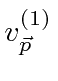and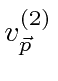that are charge conjugates of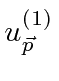and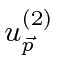.

Jim Branson 2013-04-22